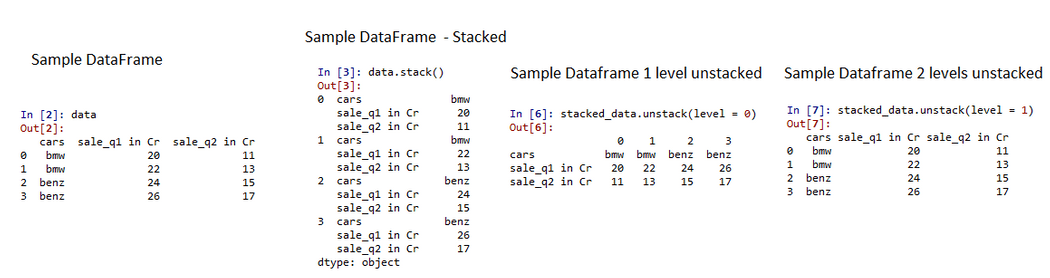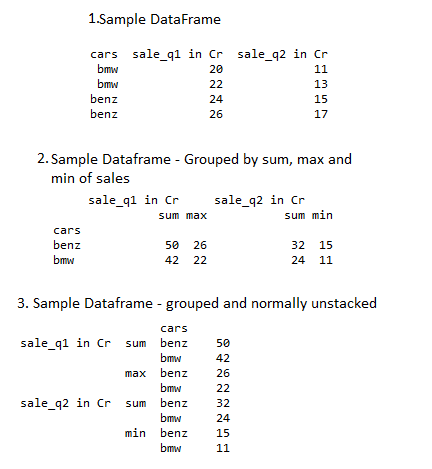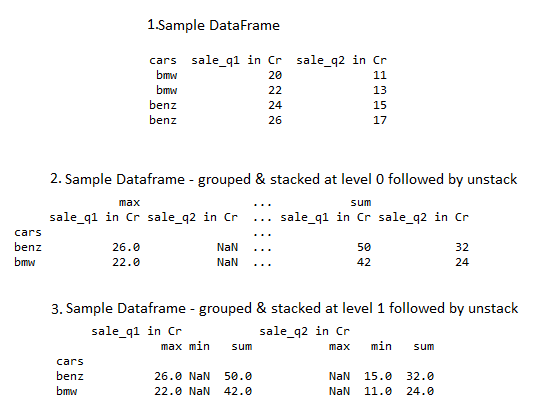# Pandas GroupBy – Unstack

• Difficulty Level : Hard
• Last Updated : 19 Jan, 2022

Pandas Unstack is a function that pivots the level of the indexed columns in a stacked dataframe. A stacked dataframe is usually a result of an aggregated groupby function in pandas. Stack() sets the columns to a new level of hierarchy whereas Unstack() pivots the indexed column. There are different ways to  Unstack a pandas dataframe which would be discussed in the below methods.

### Method 1: General Unstacking of pandas dataframe at multi-levels using unstack()

Groupby aggregation on a dataframe usually returns a stacked dataframe object, of multi-levels depending on the aggregation model.

## Python3

 `# import the python pandas package``import` `pandas as pd``# create a sample dataframe``data ``=` `pd.DataFrame({``"cars"``: [``"bmw"``, ``"bmw"``, ``"benz"``, ``"benz"``],``                     ``"sale_q1 in Cr"``: [``20``, ``22``, ``24``, ``26``],``                     ``'sale_q2 in Cr'``: [``11``, ``13``, ``15``, ``17``]},``                    ``columns``=``[``"cars"``, ``"sale_q1 in Cr"``,``                             ``'sale_q2 in Cr'``])``print``(data)` `# stack the data using stack() function``stacked_data ``=` `data.stack()``print``(stacked_data)` `# unstack the dataframe by first level``stack_level_1 ``=` `stacked_data.unstack(level``=``0``)``print``(stack_level_1)` `# unstack the dataframe by second level``stack_level_2 ``=` `stacked_data.unstack(level``=``1``)``print``(stack_level_2)`

Output:Code Explanation:

• Create a sample dataframe showing car sales in two quarters.
• Now, stack the dataframe using stack() function, this will stack the columns to row values.
• As we have two columns while unstacking it will be considered as two different levels.
• Now, use unstack function with level 0 and level 1 separately to stack the dataframe at two different levels.
• It depends on the use case to stack the first or second level.

### Method 2: GroupBy Unstacking of pandas dataframe with simple unstack()

Whenever we use groupby function on pandas dataframe with more than one aggregation function per column, the output is usually a multi-indexed column where as the first index specifies the column name and the second column index specifies the aggregation function name.

## Python3

 `# import the python pandas package``import` `pandas as pd` `# create a sample dataframe``data ``=` `pd.DataFrame({``"cars"``: [``"bmw"``, ``"bmw"``, ``"benz"``, ``"benz"``],``                     ``"sale_q1 in Cr"``: [``20``, ``22``, ``24``, ``26``],``                     ``'sale_q2 in Cr'``: [``11``, ``13``, ``15``, ``17``]},``                    ``columns``=``[``"cars"``, ``"sale_q1 in Cr"``,``                             ``'sale_q2 in Cr'``])``print``(data)` `# aggregate the car sales data by sum min``# and max sales of two quarters as shown``grouped_data ``=` `data.groupby(``'cars'``).agg(``    ``{``"sale_q1 in Cr"``: [``sum``, ``max``],``     ``"sale_q2 in Cr"``: [``sum``, ``min``]})``print``(grouped_data)` `# general way of unstacking the grouped dataframe``gen_unstack ``=` `grouped_data.unstack()``print``(gen_unstack)` `# stacking the grouped dataframe at``# different levels and unstacking``# unstacking the stacked dataframe at level = 0``unstack_level1 ``=` `grouped_data.stack(level``=``0``).unstack()``print``(unstack_level1)` `# unstacking the stacked dataframe at level =1``unstack_level2 ``=` `grouped_data.stack(level``=``1``).unstack()``print``(unstack_level2)`

Output:Code Explanation:

• Create a sample dataframe showing car sales in two quarters.
• Use GroupBy function to group the car sales data by sum min and max sales of two quarters as shown
• As we have two columns while unstacking it will be considered as two different levels at two indexes. The first index will have the column name and the second index will have the name of the aggregated function.
• Now, perform a simple unstack operation on the grouped dataframe. This simple unstack will convert the columns as rows and vice versa as shown in the output

### Method 3: GroupBy Unstacking of pandas dataframe with multiple unstack() at two different levels.

Generally, to have more depth in insights generated by GroupBy function, it is normally stacked at different levels of the grouped dataframe. This grouped dataframe can be further investigated by unstacking at different levels using unstack() function. The practical implementation is given below.

## Python3

 `# import the python pandas package``import` `pandas as pd``# create a sample dataframe``data ``=` `pd.DataFrame({``"cars"``: [``"bmw"``, ``"bmw"``, ``"benz"``, ``"benz"``],``                     ``"sale_q1 in Cr"``: [``20``, ``22``, ``24``, ``26``],``                     ``'sale_q2 in Cr'``: [``11``, ``13``, ``15``, ``17``]},``                    ``columns``=``[``"cars"``, ``"sale_q1 in Cr"``,``                             ``'sale_q2 in Cr'``])``print``(data)` `# aggregate the car sales data by sum min and``# max sales of two quarters as shown``grouped_data ``=` `data.groupby(``'cars'``).agg(``    ``{``"sale_q1 in Cr"``: [``sum``, ``max``], ``"sale_q2 in Cr"``: [``sum``, ``min``]})``print``(grouped_data)` `# stacking the grouped dataframe at``# different levels and unstacking``# unstacking the stacked dataframe at level = 0``unstack_level1 ``=` `grouped_data.stack(level``=``0``).unstack()``print``(unstack_level1)` `# unstacking the stacked dataframe at level =1``unstack_level2 ``=` `grouped_data.stack(level``=``1``).unstack()``print``(unstack_level2)`

Output:Code Explanation:

• Create a sample dataframe showing car sales in two quarters.
• Use GroupBy function to group the car sales data by sum min and max sales of two quarters as shown
• As we have two columns while unstacking it will be considered as two different levels at two indexes. The first index will have the column name and the second index will have the name of the aggregated function.
• Now, use stack() at level 0 of the grouped dataframe and unstack() the grouped dataframe.
• Then, use stack() at level 1 of the grouped dataframe and unstack() the grouped dataframe.
• It depends on the use case to unstack the dataframe at the first or second level.

My Personal Notes arrow_drop_up